Next: Toroidal Compactifications Up: SUPERSTRING PHENOMENOLOGY BEFORE 1995 Previous: SUPERSTRING PHENOMENOLOGY BEFORE 1995

## String Model Building

We mentioned in the introduction that there are only five consistent superstring theories in 10D. To build string models is the same as explicitly constructing the string vacua of each of these theories. By this we mean solutions of the corresponding background field equations of the different massless modes of the string.

Since there is no second quantized formulation of string theory, we need to use first quantization. In this case the basic quantity is the 2D worldsheet action, which for the bosonic string is: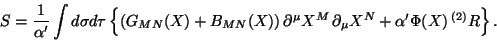(1)

Let us describe the different quantities entering into this action. First the integral is over the 2D surface swept by the movement of the string. This surface is parametrized by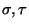. The inverse string tension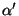is the only (constant) free parameter of the theory.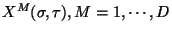play two different roles: they are scalar fields in the 2D theory, but they are coordinates of the target space where the string propagates, which for critical string theories (the subject of this paper) has dimension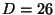. Similarly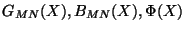are couplings of the 2D theory but since they are functions of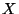they are fields in target space.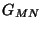is a symmetric tensor which is identified with the metric;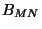is an antisymmetric tensor field which in 4D target space will give rise to an axion field; and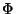is a scalar field, the dilaton. Since it appears only multiplying the 2D curvature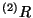whose integral is the topological invariant that counts the genus (number of holes) of the corresponding 2D surface, the vev of the dilaton is identified with the string coupling. These fields are always present in any closed string.

A fundamental symmetry of the above action is conformal invariance which includes scalings of the 2D metric as well as 2D reparametrization invariance. Imposing this symmetry at the 2D quantum level is similar to imposing that the coupling constants do not run in standard field theory. This then defines a 2D conformal field theory (CFT) and the constraints on the 2D couplings are the field equations for the target space fields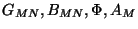. Not surprisingly the constraints give rise to Einstein's equations, Yang-Mills equations and equations of motion forand. To leading order inthese are the equations derived from the following target spacetime effective action: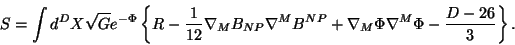(2)

Since heterotic strings are supersymmetric, we have to add the corresponding fermionic partners of those fields. Solutions of these equations are then what we call string vacua and thus we can claim that there is a correspondence between string vacua and certain CFTs in 2D.

The simplest solution is of course 26D flat spacetime with constant values of all the fields. For this case we have a 2D free theory, which can be easily quantized by solving the wave equation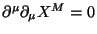, the fields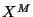can be written as: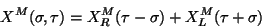(3)

as usual,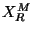and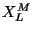represent right- and left-moving modes of the string respectively, with the mode expansion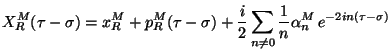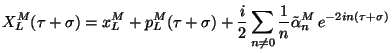(4)

Since this is a free theory, quantization assigns canonical commutation relations to the Fourier coefficients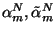, like oscillators of the harmonic oscillator. The Hamiltonian then gives rise to the mass formula: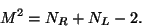(5)

Where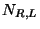refer to the harmonic oscillator occupation numbers for left and right movers and the level matching condition requires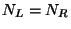for consistency. Note that the `vacuum' state (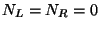) is a tachyon and the next state requires one left-moving and one right-moving oscillator (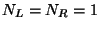), since both oscillators carry a target space index, the state corresponds to an arbitrary two-index tensor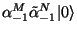of which the symmetric part is the metric, the antisymmetric part isand the trace is the dilaton. That we can see are massless and are always present.

The instability due to the tachyon can be easily cured by supersymmetrizing the theory. In that case the tachyon state is projected out. The most popular supersymmetric string theory is the heterotic string. In this theory, only the right moving modes have a fermionic partner and consistency requires that they live in a 10D space rather than the 26D space of the bosonic string. The left moving modes however are purely bosonic, but the 26D space of these modes is such that the extra 16 coordinates are toroidally compactified, giving rise to extra massless states, which in this case are vector-like, as we will see next, and correspond to the gauge fields of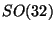or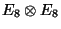.Next: Toroidal Compactifications Up: SUPERSTRING PHENOMENOLOGY BEFORE 1995 Previous: SUPERSTRING PHENOMENOLOGY BEFORE 1995
root 2001-01-22